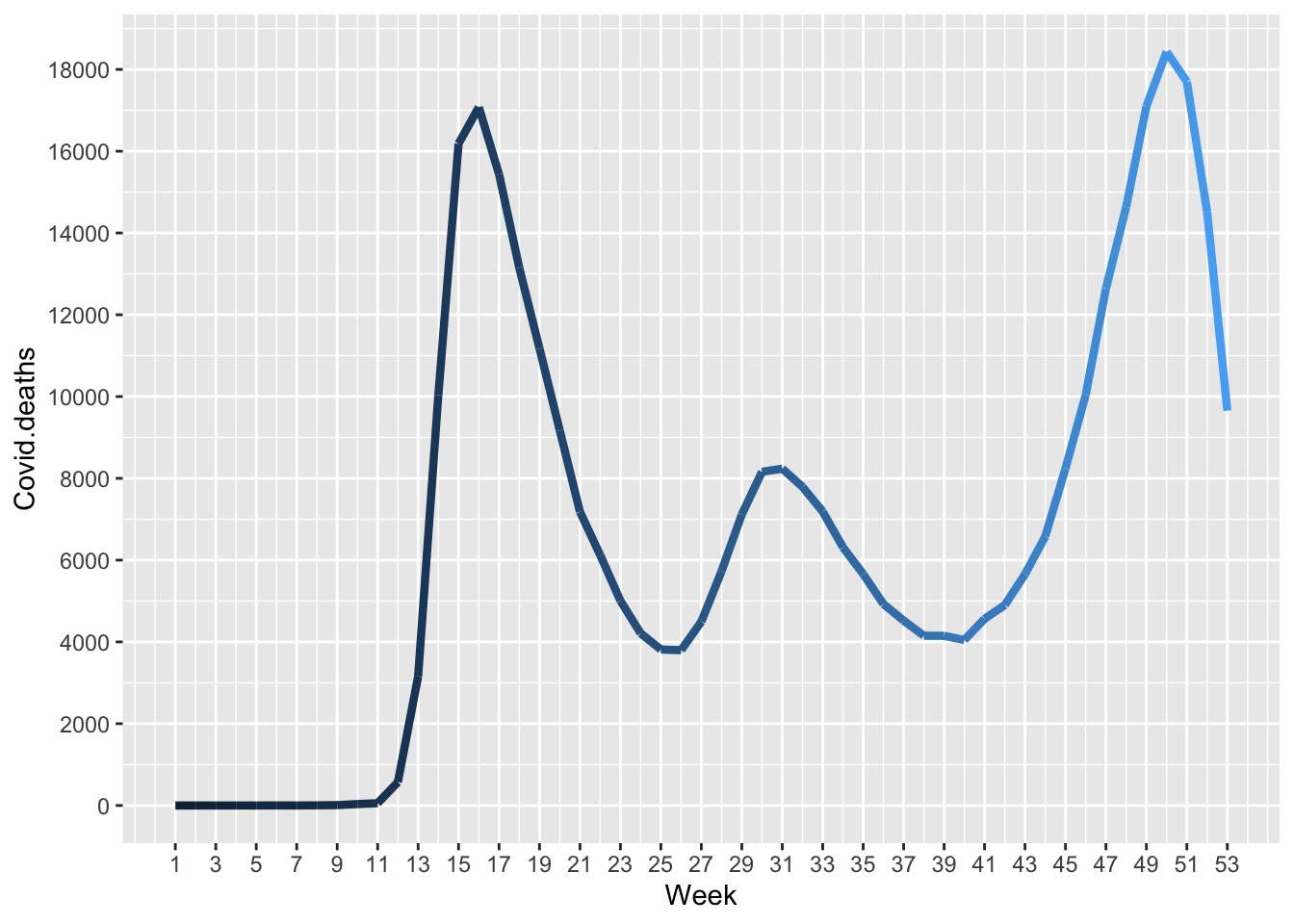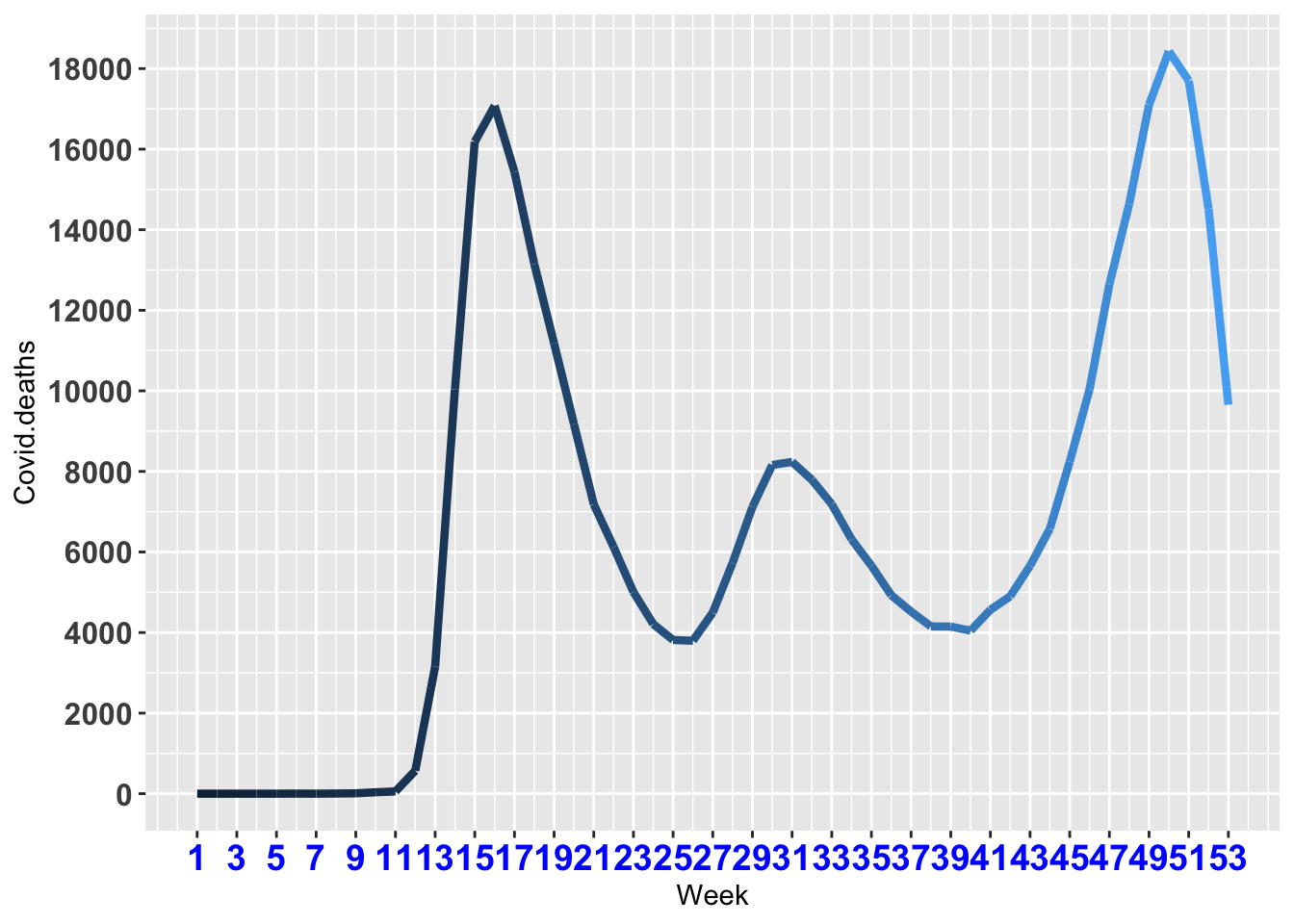# Demonstration of specification of x and y axes

# Change scale

p = ggplot(data=us, aes(x=Week, y=Covid.deaths, col=Week)) + geom_line(lwd=1.5)

p + scale_x_continuous(breaks = seq(from = 1, to = 53, by = 2)) + scale_y_continuous(breaks = seq(from = 0, to = 20000, by = 2000)) + theme(legend.position="none")# Change font and size of x & y-axis

p = ggplot(data=us, aes(x=Week, y=Covid.deaths, col=Week)) + geom_line(lwd=1.5)

p + theme(legend.position="none", axis.text.x=element_text(face="bold", size=14, color="blue", angle=0), axis.text.y=element_text(face="bold", size=12)) + scale_x_continuous(breaks = seq(from = 1, to = 53, by = 2)) + scale_y_continuous(breaks = seq(from = 0, to = 20000, by = 2000)) + theme(legend.position="none")